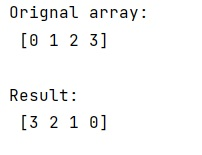# Most efficient way to reverse a NumPy array

In this tutorial, we will learn about the most efficient way to reverse a NumPy array. By Pranit Sharma Last updated : May 23, 2023

Suppose that we are given a NumPy array that contains some numerical values and we need to reverse the order of this array.

## Reversing a NumPy Array

Python has a very popular code snippet that is used for reversing an array. Reversing an array can be done by using the following code snippet:

```reverse = arr[::-1]
```

Hence, we will follow this approach to deliver the NumPy array. This method gives a reversed view of the original array.

Any changes made to the original array will also be immediately visible in the reversed array. The underlying data buffers for array and reverse an array is shared, so creating this view is always instantaneous and does not require any additional memory allocation for copying the array contents.

Let us understand with the help of an example,

## Python program for reversing a NumPy array

```# Import numpy
import numpy as np

# Creating a numpy arrray
arr = np.array([0, 1, 2, 3])

# Display original array
print("Orignal array:\n",arr,"\n")

# Reversing an array
res = arr[::-1]

# Display Result
print("Result:\n",res,"\n")
```

Output: